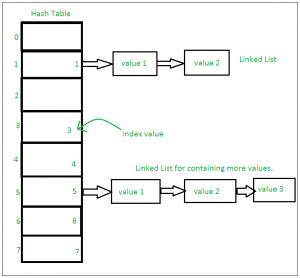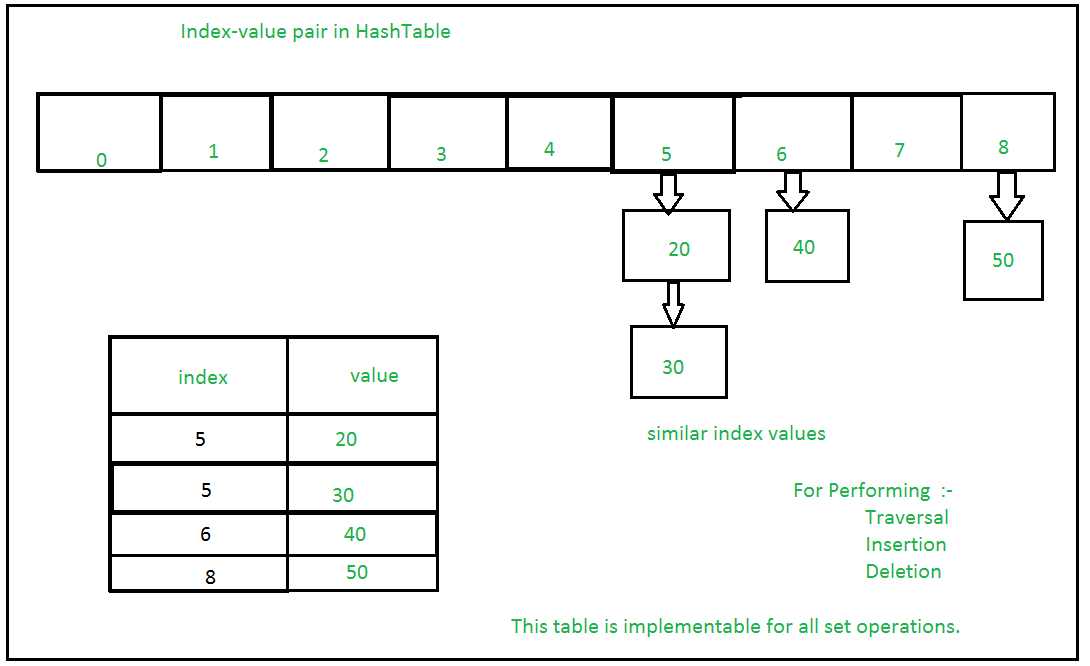# Internal working of Set in Python

Sets and their working
Set in Python can be defined as the collection of items. In Python, these are basically used to include membership testing and eliminating duplicate entries. The data structure used in this is Hashing, a popular technique to perform insertion, deletion and traversal in O(1) on average. The operations on Hash Table are some what similar to Linked List. Sets in python are unordered list with duplicate elements removed.

Basic Methods on Sets are:-
Creating Set:- In Python, Sets are created through set() function. An Empty list is created. Note that empty Set cannot be created through {}, it creates dictionary.

Checking if an item is in : Time complexity of this operation is O(1) on average. However u=in worst case it can become O(n).

Adding elements:- Insertion in set is done through set.add() function, where an appropriate record value is created to store in the hash table. Same as checking for an item, i.e., O(1) on average. However u=in worst case it can become O(n).

Union:- Two sets can be merged using union() function or | operator. Both Hash Table values are accessed and traversed with merge operation perform on them to combine the elements, at the same time duplicates are removed. Time Complexity of this is O(len(s1) + len(s2)) where s1 and s2 are two sets whose union needs to be done.

Intersection:- This can be done through intersection() or & operator. Common Elements are selected. They are similar to iteration over the Hash lists and combining the same values on both the Table. Time Complexity of this is O(min(len(s1), len(s2)) where s1 and s2 are two sets whose union needs to be done.

Difference:- To find difference in between sets. Similar to find difference in linked list. This is done through difference() or – operator. Time complexity of finding difference s1 – s2 is O(len(s1))

Symmetric Difference:- To find element in both the sets except the common elements. ^ operator is used. Time complexity of s1^s2 is O(len(s1))

Symmetric Difference Update: Returns a new set which contains symmetric difference of two sets. Time complexity is O(len(s2))

clear:- Clears the set or Hash Table.

Time complexity source : Python Wiki

If Multiple values are present at the same index position, then the value is appended to that index position, to form a Linked List. In, CPython Sets are implemented using dictionary with dummy variables, where key beings the members set with greater optimizations to the time complexity.

Set Implementation:-Sets with Numerous operations on a single HashTable:-Examples:

```# empty set, avoid using {} in creating set or dictionary is created
x = set()

# set {'e', 'h', 'l', 'o'} is created in unordered way
B = set('hello')

# set{'a', 'c', 'd', 'b', 'e', 'f', 'g'} is created
A = set('abcdefg')

# set{'a', 'b', 'h', 'c', 'd', 'e', 'f', 'g'}

fruit ={'orange', 'banana', 'pear', 'apple'}

# True  fast membership testing in sets
'pear' in fruit

'mango' in fruit     # False

A == B       # A is equivalent to B

A != B       # A is not equivalent to B

A <= B    # A is subset of B A <B>= B

A > B     # A is proper superset of B

A | B        # the union of A and B

A & B     # the intersection of A and B

A - B        # the set of elements in A but not B

A ˆ B        # the symmetric difference

a = {x for x in A if x not in 'abc'}   # Set Comprehension
```
My Personal Notes arrow_drop_upIf you like GeeksforGeeks and would like to contribute, you can also write an article using contribute.geeksforgeeks.org or mail your article to contribute@geeksforgeeks.org. See your article appearing on the GeeksforGeeks main page and help other Geeks.

Please Improve this article if you find anything incorrect by clicking on the "Improve Article" button below.

Improved By : COMMITMENT

Article Tags :
Practice Tags :

2

Please write to us at contribute@geeksforgeeks.org to report any issue with the above content.